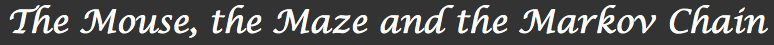1. Introduction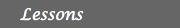2. The mouse and the maze 3. Processes, matrices and Markov chains 4. Will the mouse ever find the exit? How fast? 5. Other examples and applicationsProcesses, matrices and Markov chains

The kind of process we described in the previous section is an example of a very general kind of processes called Markov chains. To describe such a Markov chain, we need the following data:

First, we have a set of states, S = {s1, s2, ... , sr }. The process starts in one of these states and moves successively from one state to another, in the very same way our mouse moves from room to room in the maze. Each move is called a step.

If the chain is currently in the state si, then it moves to the state sj at the next step with a probability denoted by pji , and this probability does not depend upon which states the chain was in before the current state. The probabilities pji are called, once again, transition probabilities. While we did not allow it in our first example, in general, the process can remain in the state it is in, and this occurs with probability pii.

An initial probability distribution, defined on S, specifies the starting state. Usually this is done by specifying a particular state as the starting state. More generally, this is given by the distribution of probabilities of starting in each of the states. For example, in the 5-state process describing our mouse, the distribution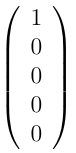would mean that the mouse is in the first room at the beginning of the experiment, while the statewould represent the starting state of the mouse in the unfair 5-sided die experiment.

We can represent the process with a graph whose vertices (nodes) represent the different states the system can be in, while the arrows represent the possible steps. Over each arrow we write the transition probability associated with that step.

Question
Going back to the mouse example, the figure below is part of the graph representing the mouse process. Can you complete it?Such a graph makes it easy to visualize the process, but what really makes Markov chains a computationally efficient framework is the matrix representation.

We write the transition probabilities in an array as follows: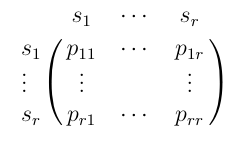This means that to find the transition probability from state i to state j, we look up the initial state on the top line and the new state on the left. The number at the intersection of that row and that column and the transition probability we’re looking for.

Question
Write the transition matrix for Little Ricky in the maze.

The power of this framework comes to light when one realizes that equations (3) and (1) are nothing but a rewriting of the multiplication rule for vectors and matrices. In summary if we rewrite what we showed in the previous section using this matrix language, we can prove the following theorem:

Theorem: Fundamental Theorem of Markov Chains
If P = (pij) is the transition matrix for a Markov chain and q = (q1...qn)t is a distribution of probabilities on the states of that chain then:
• (i) The distribution of probability values on the states after 1 step starting on s is given by the matrix-vector product P q where the ith component of the new vector is given by: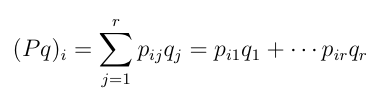• (ii) The probability of ending up in state j after 2 steps starting from state i is given by (P2)ji where P denotes the product of the matrix P with itself. Explicetely: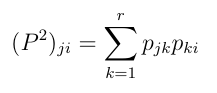• (ii’) More generally, the transition probabilities after n steps are given by the transition matrix Pn, the n-fold product of the matrix P with itself.